# AP Board 4th Class Maths Solutions 6th Lesson Division

Andhra Pradesh AP Board 4th Class Maths Solutions 6th Lesson Division Textbook Exercise Questions and Answers.

## AP State Syllabus 4th Class Maths Solutions Chapter 6 Division

Textbook Page No. 71

Think and Discuss

For a fixed number of papads, if the number of papads in a packet in* creases, then the number of packets.
Solution:
If the number of papads in a packet increases, then the number of packets decreases.

Textbook Page No. 71

Do this

a) If 108 pencils are packed in 9 boxes, then find the number of pencils in each box.
Solution:
Number of pencils = 108
Number of boxes = 9
Number of pencils in each box = 108 ÷ 9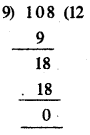= 12 pencilsb) Kiran arranged 168 chairs from 6 rows equally. How many chairs will be in each row ?
Solution:
Number of chairs = 168
Number of rows = 6
Number of chairs in each row = 168 ÷ 6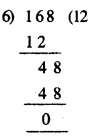= 28 chairs.

Textbook Page No. 73

Do this

1. Find the quotient and remainder in the following divisions.

a) 808 ÷ 8
Solution:
808 ÷ 8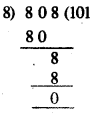∴ Quotient = 101
Remainder = 0

b) 996 ÷ 6
Solution:
996 ÷ 6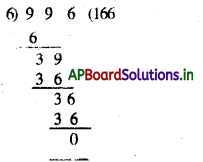∴ Quotient = 166
Remainder = 0

c) 408 ÷ 3
Solution:
408 ÷ 3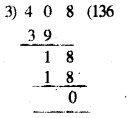∴ Quotient = 136
Remainder = 0Question 2.
A fruit seller packed 108 custard apples in 9 baskets. How many custard apples did each basket hold ?
Solution:
Number of custard apples = 108
Number of custard apples in a basket = 108 ÷ 9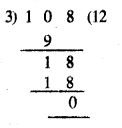= 12 apples

Textbook Page No. 75

Do this

1. Divide and check the result.

a) 509 ÷ 9
Solution: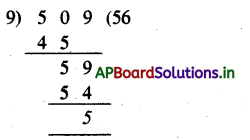Dividend = 509
Divisor = 9
Quotient = 56
Remainder = 5

Rule:
Dividend = (Divisor × Quotient) + Remainder
509 = (9 × 56) + 5
509 = 504 + 5 = 509b) 721 ÷ 8
Solution: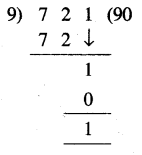Dividend = 721
Divisor = 8
Quotient = 90
Remainder = 1

Rule:
Dividend = (Divisor × Quotient) + Remainder
721 = (8 × 90) + 1
721 = 720 + 1 = 721

2. Find the quotient and remainder in the following division problems.

a) 479 ÷ 8
Solution: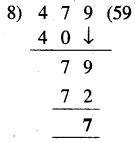Quotient = 59
Remainder = 7b) 983 ÷ 5
Solution: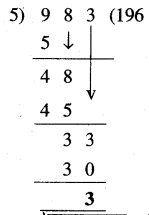Quotient = 59
Remainders = 7

c) 843 ÷ 3
Solution: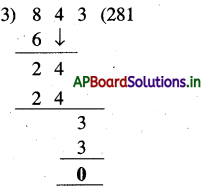Quotient = 281
Remainder = 0

Try this

Question 1.
240 and 176 are divisible by 16. Can their difference also be divisible by 16 ?
Solution:
Difference = 240 – 176
= 64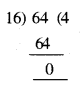64 ÷ 16 = 4
∴ Difference also be divisible by 16Question 2.
Divide 180 by 1,2,3,4,5 and 6. What do you observe ?
Solution:
Dividend = 180
Divisors = 1, 2, 3, 4, 5 and 8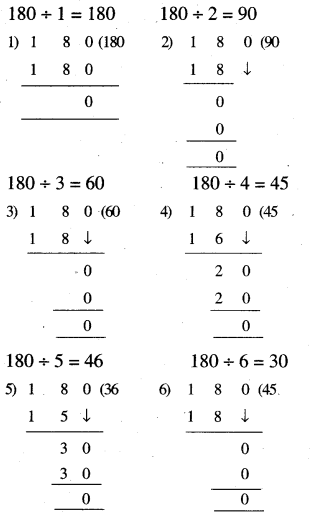Observation :
180 is divided by 1, 2, 3, 4, 5 and 6 exactly In each case 1 got remainder is ‘0’.

Exercise – 6.1

Question 1.
If the cost of each pen is ₹ 6, then how many pens can we get for ₹ 864 ?
Solution:
Cost of each pen = 6
Amount we have = 864
Number of pens we get = 864 ÷ 6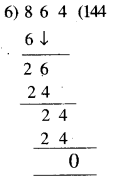= 144 pensQuestion 2.
8 pupils went went to circus and they paid ₹ 360 for tickets. What is the cost of each ticket ?
Solution:
Number of pupils went to circus = 8
Paid amount to tickets = 360

Question 3.
One sheet of brown paper is needed to cover 6 note books. How many brown sheets are required to cover 114 such books ?
Solution:
Number of notebooks covered by brown sheet = 6
Total books we have = 114
Number of brown sheets required = 114÷ 6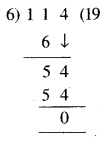= 19 sheets

4. Fill the boxes with suitable number.

a)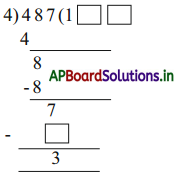Solution: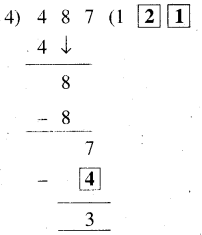b)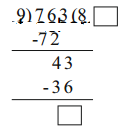Solution: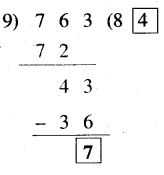Question 5.
A drum has 500 litres of water. How many 20 litre cans can be filled with water ?
Solution:
Capacity of drums = 500 ltrs
Capacity of can = 20 ltrs
Number of cans required 500 ÷ 20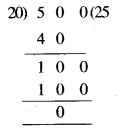= 25 cansQuestion 6.
Total bus fare from Vijayanagaram to Visakhapatnam for 9 people is 540. What is the bus fare for each person ?
Solution:
Number of people in the bus = 9
Total fare from Vijayanagaram to Visakhapatnam = ₹ 540
Bus fare for each person = ₹ 540 ÷ 9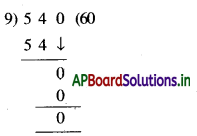= ₹ 60

Question 7.
183 ÷ 9 Rakesh did this problem like this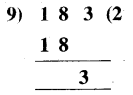Quotient = 2
Remainder = 3
Solution:
No, Rakesh is wrong.
Justification: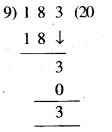∴ Quotient = 20
Remainder = 3

Textbook Page No. 78

Do this

1. Find the quotient and the remainder for the following and check your answer.

a) 309 ÷ 15
Solution: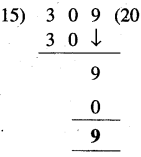Quotient = 9
Remainder = 20

Checking:
Dividend = Divisor × Quotient + Remainder
309 = 15 × 20 + 9
= 300 + 9 = 309b) 768 ÷ 19
Solution: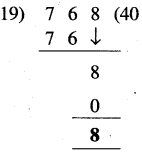Quotient = 40
Remainder = 8

Checking:
Dividend = Divisor × Quotient + Remainder
768 = 19 × 40 + 8
= 760 + 8 = 768

c) 422 ÷ 24
Solution: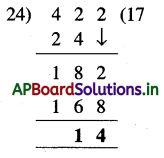Quotient = 7
Remainder = 14

Checking :
Dividend = Divisor × Quotient + Remainder
422 = 24 × 17 + 14
= 408 + 14 = 422

d) 849 ÷ 42
Solution: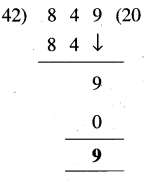Quotient = 20
Remainder = 9

Checking:
Dividend = Divisor × Quotient + Remainder
849 = 42 × 20 + 9
= 840 + 9 = 849Question 2.
A garment vendor packs 24 T-shirts in a box. If he had 886 T-shirts, how many boxes are required to pack and how many T-shirts would remain unpacked ?
Solution:
Number of T-shirts in a box = 24
Total T.shirts = 886
Number of boxes required = 886 ÷ 24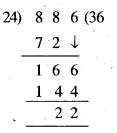= 22 boxes
Number of unpacked T-shirts = 22

Textbook Page No. 80

Do this

Guess the remainder and quotient without actual division.

a) 649 ÷ 10
Solution:
649 ÷ 10 = 600
Quotient = 600
Remainder = 49

b) 989 ÷ 100
Solution:
989 ÷ 100 = 900
Quotient = 900
Remainder = 89c) 701 ÷ 100
Solution:
701 ÷ 100 = 700
Quotient = 700
Remainder = 1

d) 683 ÷ 100
Solution:
683 ÷ 100
Quotient = 600
Remainder = 83

Exercise – 6.2

Question 1.
Dasu needs 3 oranges to make a glass of orange juice. How many glasses of orange juice can be make with 240 oranges ?
Solution:
Number of organges required to make a glass of juice = 3
Number of oranges we have = 240
Number of glasses will make = 240 ÷ 3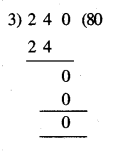= 80 glassesQuestion 2.
The cost of a mango is ₹ 15. How many mangoes can be purchased for ₹ 210 ?
Cost of a mango is = ₹ 15
Money we have = ₹ 210
Number of mangoes we can be purchased = 210 ÷ 15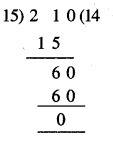= 14 mangoes

Question 3.
The earth takes 24 hours to complete One rotation. How many rotations can it make in 144 hours ?
Solution:
Number of hours to complete one rotation = 24 hrs
Number of hours we have = 144 hrs
Number of rotations = 144 ÷ 24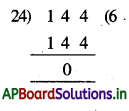= 6 rotations

Question 4.
A school bus can accommodate 50 children. How many such buses are needed to accommodate 250 children ?
Solution:
Capacity of school bus = 50 children
Number of children = 250
Number of buses required = 250 ÷ 50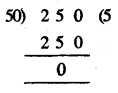= 5 busesQuestion 5.
160 children get into teams of 4. How many such teams can they form ?
Solution:
No. of children in each team = 4
Number of children in Total = 160
Number of teams = 160 ÷ 4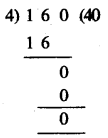= 40 teams

Question 6.
How many weeks make 126 days ?
Solution:
Number of days in a week = 7
Number of days in week = 126
Number of weeks = 126 ÷ 7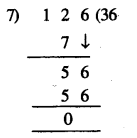= 18 weeksQuestion 7.
Sanju bought 360 crayons in packets of 15 each. How many packets of crayons did Sanju buy ?
Solution:
Number of crayons in each packet = 15
Sanju have crayons = 360
Number of packets Sanju bought
= 360 ÷ 5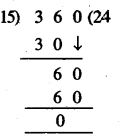= 24 packets

8. Fill in the missing digits in the division and find the quotient and the remainder.

a)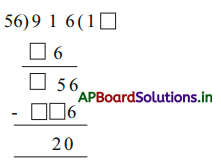Quotient = ____________
Remainder = ___________
Solution: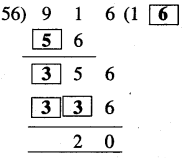Quotient = 16
Remainder = 20

b)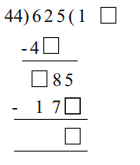Quotient = ____________
Remainder = ___________
Solution:Quotient = 14
Remainder = 9Question 9.
Rani did the problem in way.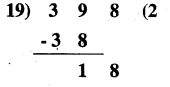Quotient = 2
Remainder = 18
Is it correct ? Justify.
Solution:
It is wrong.
Justification: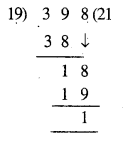Quotient = 21
Remainder = 1

Question 10.
A small scale industry made 750 candles in a week. The candles were packed in a packet of 12 each. How many packets were made and how many candles were left behind ?
Solution:
Number of candles made in a week = 750
Number of candles packed in a packet = 12
Number of candles were made = 750 ÷ 12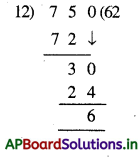= 62 packets
∴ Number of candles left = 6

Textbook Page No. 83

Try this

7 + 7 + 7 + 7 × 7 – 7
Solution:
Given that 7 + 7 ÷ 7 + 7 × 7 – 7
By applying “DMAS” rule
= 7 + 1 + 7 × 7 – 7 {∵ 7 ÷ 7 = 1}
= 7 + 1 + 49 – 7 {∵ 7 × 7 = 49}
= 57 – 7 {∵ 7+ 1 + 49 = 57}
= 50

Exercise – 6.3

Question 1.
168 ÷ 8 + 5 × 12 – 38
Solution:
Given that 168 ÷ 8 + 5 × 12 – 38
By applying “DMAS” rule
= 21 + 5 × 12    {∵ 168 ÷8 = 21}
= 21 + 60 = 81   {∵ 5 × 12 = 60}Question 2.
412 – 108 + 315 ÷ 45 × 157
Solution:
Given that 412 – 108 + 315 ÷ 45 × 157
By applying “DMAS” rule
= 412 – 108 + 7 × 157   {∵ 315 ÷ 45 = 7}
= 412 – 108+ 1099    {∵ 412 + 1099 = 1511}
= 1511 – 108    {∵ 1511 – 108 = 1403}
= 1403

Question 3.
476 ÷ 14 × 24 – 504 + 132
Solution:
Given that 476 ÷ 14 × 24 – 504 + 132
By applying “DMAS” rule
= 34 × 24 – 504 + 132   {∵ 476 ÷ 14 = 34}
= 816 – 504 + 132   {∵ 34 × 24 = 816}
= 948 – 504    {∵ 816+ 132 = 948}
= 444

Question 4.
482 – 412 + 276 ÷ 12 × 204
Solution:
Given that 482 – 412 + 276 ÷ 12 × 204
By applying “DMAS” rule
482 – 412 + 276 ÷ 12 × 204   {∵ 276 ÷ 12 = 23}
= 482 – 412 + 23 + 4692    {∵ 23 × 204 = 4693}
= 5197 – 412
= 4785Question 5.
128 + 125 ÷ 25 × 26 – 127
Solution:
Given that 128 + 125 ÷ 25 × 26 – 127
By applying “DMAS” rule
128 + 5 × 26 – 127 {∵ 125 + 25 = 5}
= 128 + 130 – 127 {∵ 5 × 26 = 130}
= 258 – 127
= 131

Multiple Choice Questions

Question 1.
In 602 ÷ 5, divisor is
A) 120
B) 602
C) 5
D) All
C) 5

Question 2.
Divide 480 by 10, Quotient is
A) 47
B) 48
C) 0
D) 480
B) 48Question 3.
In 384 ÷ 6, dividend is
A) 6
B) 384
C) 64
D) All
B) 384

Question 4.
Dividend = Divisor × __________ + Remainder
A) Quotient + 2
B) Quotient × 2
C) Quotient
D) Quotient ÷ 2
C) Quotient

Question 5.
In 598 ÷13, Remainder is
A) 0
B) 13
C) 8
D) None
A) 0

Question 6.
One apple costs ₹ 24. How much does 20 apples cost ?
A) 400
B) 480
C) 250
D) 240
B) 480Question 7.
There are 18 Balloons. If we give 6 balloons to each child, to how many children can we give these balloons ?
A) 18
B) 6
C) 3
D) 0
C) 3

Question 8.
Divide 15 books is equal groups of 5
A) 3
B) 2
C) 5
D) 4
A) 3

Question 9.
Repeated subtraction is called
A) Multiplication
B) Division
D) Subtraction